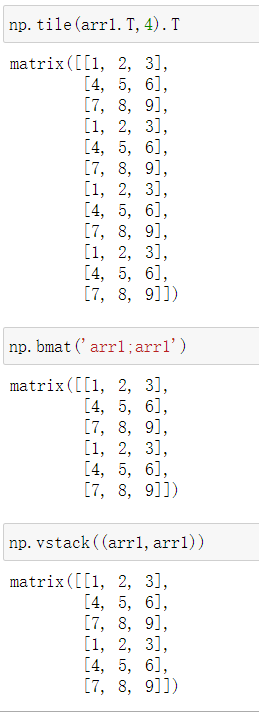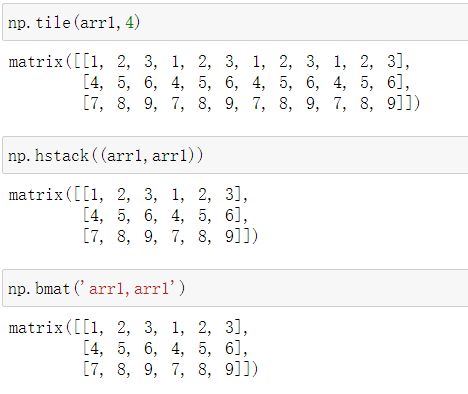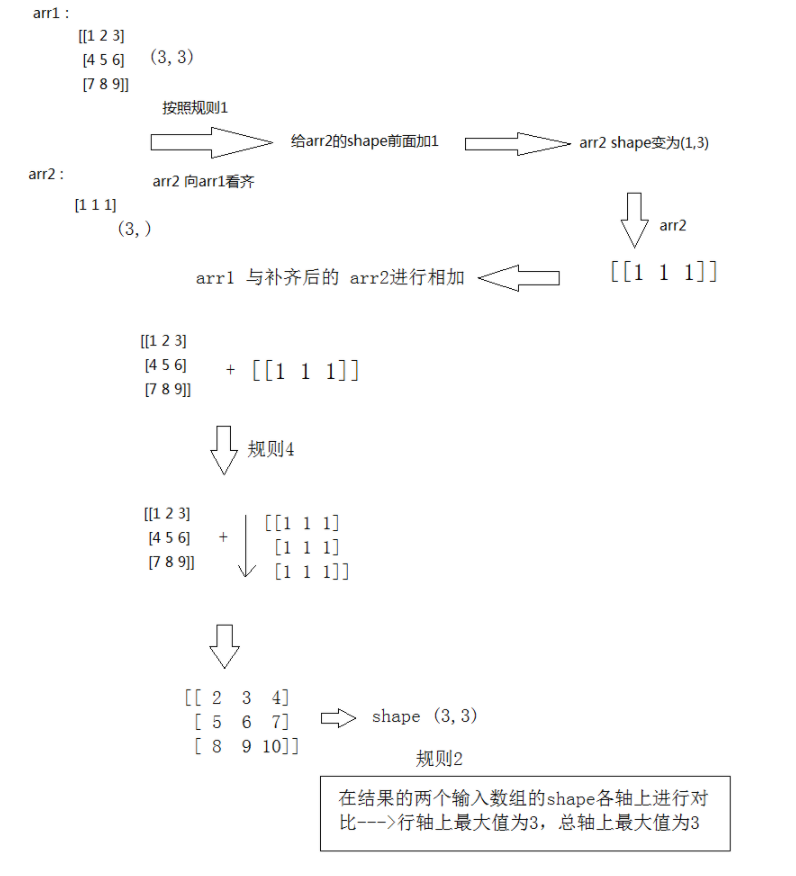• 纵向复制 横向复制
纵向复制横向复制展开全文• repeat函数功能：对数组中的元素进行连续重复复制 用法有两种： 1) numpy.repeat(a, repeats, axis=None) 2) a.repeat(repeats, axis=None)   self.user_emb.shape Out: (512,) 目标shape（13214， 512...
repeat函数功能：对数组中的元素进行连续重复复制
用法有两种：
1) numpy.repeat(a, repeats, axis=None)
2) a.repeat(repeats, axis=None)

self.user_emb.shape
Out: (512,)

目标shape（13214， 512）

#先增加一个维度

user_emb_dims = np.expand_dims(self.user_emb, axis=0)
user_emb_dims.shape
Out: (1, 512)

#然后再对矩阵进行重复
user_emb_arr = np.repeat(user_emb_dims, 13214, axis=0)
user_emb_arr.shape
Out: (13242, 512)

# 时间 0.017989158630371094

# 方法二：
user_emb_arr2 = user_emb_dims.repeat(13242, axis=0)
时间： 0.01998972892761230
tile函数功能：对整个数组进行复制拼接
用法：numpy.tile(a, reps)
其中a为数组，reps为重复的次数
user_emb_arr4 = np.tile(user_emb_dims,(13242,1))

时间： 0.020986557006835938

>>> a=np.array([10,20])
>>>a
array([10,20])
>>>np.tile(a, (3,2)) #构造3*2个copy
array([[10, 20, 10, 20],
[10, 20, 10, 20],
[10, 20, 10, 20]])
---------------------
作者：悟乙己
来源：CSDN
原文：https://blog.csdn.net/sinat_26917383/article/details/52290505
版权声明：本文为博主原创文章，转载请附上博文链接！
参考文章：https://blog.csdn.net/sinat_26917383/article/details/52290505
展开全文• 矩阵numpy.matrix类类型的对象 该类继承自numpy.ndarray 任何针对多维数组的操作，对矩阵同样有效 但是作为子类矩阵又结合其自身的特点，做了必要的扩充，比如：乘法计算(行*列)、求逆等。 矩阵的创建 #方法1...
矩阵是numpy.matrix类的对象 该类继承自numpy.ndarray,所以任何针对多维数组的操作，对矩阵同样有效 但是作为子类矩阵又结合其自身的特点，做了必要的扩充， 比如：乘法计算(行*列)、求逆等。
矩阵的创建
#方法1
numpy.matrix(ary,copy=True)
#参数:
#ary：任何可被解释为矩阵的二维容器(经常为二维数组)
#copy:是否复制数据(缺省值为True时复制数据，与原容器相互独立)

#方法2
numpy.mat(任何可被解释为矩阵的二维容器)
#等价于numpy.matrix中copy=False,与原容器共享数据，不拥有独立的书拷贝（改一个另一个也改了)

#方法3
#该函数可以接受字符串形式的矩阵描述
#数据项通过空格分隔，数据行通过分号分隔，如：'1 2 3; 4 5 6'
numpy.mat(拼块规则)

示例
import numpy as np

ary = np.arange(1,9).reshape(2,4)
print(ary,type(ary))
#matrix复制出独立数据空间
m1 = np.matrix(ary)
print(m1,type(m1))	#type为numpy.matrix
#mat 共享数据空间
m2 = np.mat(ary)
print(m2,type(m2))
#字符串拼块规则
print(np.mat('1 2 3; 4 5 6'))	#输出[[1 2 3] [4 5 6]]

矩阵的乘法运算
矩阵的乘法：矩阵A的每一行上的元素与对应的矩阵B的列上的元素依次相乘再相加(点积)，所以矩阵A的行数要与矩阵B的列数一样，得到的新矩阵的shape为：(矩阵A的行数，矩阵B的列数)
numpy提供了方法让多维数组使用矩阵的运算
a = np.array([
[1,2,6],
[3,5,7],
[4,8,9]
])
#点乘法求ndarray的点乘结果，与矩阵的乘法运算结果相同
k = a.dot(a)
#linalg模块中的	inv方法求a的逆矩阵
l = np.linalg.inv(a)

矩阵的逆矩阵
若两个矩阵A,B满足：AB = BA = E(E为单位矩阵,其他矩阵与单位矩阵相乘还是自身，相当于和1相乘),则称A,B为逆矩阵
#创建矩阵
e = np.mat('1 2 6;3 5 7;4 8 9')
print(e.I)	#获得逆矩阵(不是所有矩阵都有逆矩阵)
print(e * e.I)	#E单位矩阵

矩阵的应用
求斐波那契数列
f = np.mat('1 1; 1 0')
for i in range(1,20):
print(f ** i, '<-',i)	#求得的矩阵都是符合斐波那契数列的

展开全文• import numpy as np # 可以使用np.mat np.asmatrix np.bmat 来创建矩阵 # np.mat 和 np.asmatrix 是相同 # 可以将特殊字符串转化为矩阵 m1 = np.mat('1 2 3;4 5 6;7 8 9') print('m1:\n', m1) print('m1 类型：\n',...
一、矩阵创建
1、使用 np.mat、np.asmatrix 创建矩阵
np.mat 和 np.asmatrix 是相同的
代码实现：
import numpy as np

# 可以使用np.mat  np.asmatrix  np.bmat 来创建矩阵
# np.mat  和 np.asmatrix 是相同
# 可以将特殊字符串转化为矩阵
m1 = np.mat('1 2 3;4 5 6;7 8 9')
print('m1:\n', m1)
print('m1 类型：\n', type(m1))  # <class 'numpy.matrix'>

# 也可以将列表嵌套转化为矩阵
m1 = np.mat([[1, 2, 3], [4, 5, 6], [7, 8, 9]])
print('m1:\n', m1)
print('m1 类型：\n', type(m1))  # <class 'numpy.matrix'>

# 也可以将二维数组转化为矩阵
m1 = np.mat(np.array([[1, 2, 3], [4, 5, 6], [7, 8, 9]]))
print('m1:\n', m1)
print('m1 类型：\n', type(m1))  # <class 'numpy.matrix'>

# 也有属性
print('m1 的 维度：', m1.ndim)  # 矩阵的维度只能是2维
print('m1  的形状：', m1.shape)
print('m1 的元素个数：', m1.size)
print('m1 的元素的类型：', m1.dtype)
print('m1 的单个元素的大小：', m1.itemsize)

2、使用 np.bmat 来组合矩阵
代码实现:
# 使用np.bmat 来创建矩阵 ---组合矩阵
# 可以使用bmat 将二维数组转化为矩阵
m1 = np.bmat(np.array([[1, 2, 3], [4, 5, 6], [7, 8, 9]]))
print('m1:\n', m1)
print('m1 类型：\n', type(m1))

# m1 = np.bmat([[1, 2, 3], [4, 5, 6], [7, 8, 9]])  # 错误的  # np.bmat不可以将列表嵌套转化为矩阵
# m1 = np.bmat('1 2 3;4 5 6;7 8 9')  # 错误的 # np.bmat不能将特殊字符串转化为矩阵

# 组合大矩阵
# 创建两个小数组
arr1 = np.zeros((2, 2))
arr2 = np.ones((2, 2))
print('arr1:\n', arr1)
print('arr2:\n', arr2)
# 组合
m1 = np.bmat('arr1 arr2;arr2 arr1')
print('m1:\n', m1)
print('m1 的类型:\n', type(m1))

# 矩阵的索引 ---在矩阵里面索引的时候使用下标不降低维度
# 矩阵的索引 ---索引之后的结果还是矩阵  ---2维的
print('获取m1 的里面第0 行元素：', m1[0, :])

二、矩阵运算及矩阵属性
1、矩阵相加减
代码实现：
import numpy as np

# 创建矩阵
m1 = np.mat([[1, 3], [2, 1]])
m2 = np.asmatrix([[1, 2], [5, 6]])
print('m1:\n', m1)
print('m1 类型:\n', type(m1))
print('m2:\n', m2)
print('m2 类型:\n', type(m2))

# 矩阵的相加减
# 1、同型矩阵  2、矩阵对应位置元素相加减
print('m1 + m2:\n', m1 + m2)
print('m1 - m2:\n', m1 - m2)

2、矩阵与数相乘
代码实现：
import numpy as np

# 创建矩阵
m1 = np.mat([[1, 3], [2, 1]])
m2 = np.asmatrix([[1, 2], [5, 6]])
print('m1:\n', m1)
print('m1 类型:\n', type(m1))
print('m2:\n', m2)
print('m2 类型:\n', type(m2))
# 矩阵与数相乘
# 矩阵对应位置元素 与数进行相乘
print('m1 * 3 :\n', m1 * 3)

3、矩阵相乘
代码实现：
import numpy as np

# 创建矩阵
m1 = np.mat([[1, 3], [2, 1]])
m2 = np.asmatrix([[1, 2], [5, 6]])
print('m1:\n', m1)
print('m1 类型:\n', type(m1))
print('m2:\n', m2)
print('m2 类型:\n', type(m2))
# 矩阵与矩阵相乘
# 左矩阵的列 = 右矩阵的行，才能进行相乘 ---->生成一个左矩阵行、右矩阵列的矩阵
# m1 --->(2,2)
# m2 --->(2,2)
print('矩阵相乘：\n', m1 * m2)
print('矩阵相乘：\n', np.matmul(m1, m2))
print('矩阵相乘：\n', np.dot(m1, m2))

# 矩阵对应位置元素相乘
# # 1、同型矩阵 2、对应位置元素相乘
print('矩阵对应位置元素相乘：\n', np.multiply(m1, m2))

矩阵特有属性:
属性说明T返回自身的转置 matr1.TH返回自身的共轭转置 matr1.HI返回自身的逆矩阵 matr1.IA返回自身数据的 2 维数组的一个视图 matr1.A
代码实现：
import numpy as np

# 创建矩阵
m1 = np.mat([[1, 3], [2, 1]])
m2 = np.asmatrix([[1, 2], [5, 6]])
print('m1:\n', m1)
print('m1 类型:\n', type(m1))
print('m2:\n', m2)
print('m2 类型:\n', type(m2))
# 矩阵的性质
# 矩阵的转置 ---行、列互换
print('矩阵的转置：\n', m1.T)

# 矩阵的逆  -->数学里面--方阵
# 如果可逆---才能求出逆 ---不然报错或者出现警告
print('矩阵的逆：\n', m1.I)
# # 矩阵 * 矩阵的逆  = E
print('矩阵*矩阵的逆：\n', np.matmul(m1, m1.I))

# 创建一个矩阵
m3 = np.mat('1 2 3;4 1 2')
print('m3:\n', m3)
#
print('矩阵的逆：\n', m3.I)
print('矩阵* 矩阵的逆：\n', np.matmul(m3, m3.I))

# 矩阵的共轭转置 ---先共轭再转置
print('m1 的共轭转置：\n', m1.H)

# 可以通过np.mat　np.asmatrix将数组转化为矩阵
# 也可以通过 矩阵.A 将矩阵转化为数组
# 矩阵的视图
print('矩阵的视图：\n', m1.A)
print('矩阵的视图：\n', type(m1.A))  # <class 'numpy.ndarray'>

三、数组的全通用函数
全称通用函数（universalfunction），是一种能够对数组中所有元素进行操作的函数，以 Numpy 数组作为输出，因此不需要对数组每个元素都操作，比math库中的函数操作效率更高
四则运算： 加（+）、减（-）、乘（*）、除（/）、幂（**），数组间的四则运算表示对每个数组中的元素分别进行四则运算，所以形状必须相同
代码实现：
import numpy as np

# 全通用函数 ---对数组中的每一个元素都进行运算的函数
# 以整个数组为对象的一起运算 --->输出数组

# 要求：同型数组
# 创建数组
arr1 = np.array([[1, 2], [4, 3]])
arr2 = np.array([[2, 4], [2, 1]])
print('arr1:\n', arr1)
print('arr2:\n', arr2)
print('*' * 100)

# 1、四则运算 ---+ - * / **  # -----> 对应位置元素进行运算
print('数组相加：\n', arr1 + arr2)  # 对应位置元素进行相加
print('数组相减：\n', arr1 - arr2)  # 对应位置元素进行相减
print('数组相乘：\n', arr1 * arr2)  # 对应位置元素进行相乘
print('数组相除：\n', arr1 / arr2)  # 对应位置元素进行相除
print('数组相幂：\n', arr1 ** arr2)  # 对应位置元素进行求幂

比较运算： >、<、==、>=、<=、!=，比较运算返回的结果是一个布尔数组，每个元素为每个数组对 应元素的比较结果
代码实现：
# 要求：同型数组
# 创建数组
arr1 = np.array([[1, 2], [4, 3]])
arr2 = np.array([[2, 4], [2, 1]])
print('arr1:\n', arr1)
print('arr2:\n', arr2)
print('*' * 100)
# 2、比较运算 --- < > <= >= == !=  # ---->对应位置进行比较，返回的是bool数组
print('arr1 >= arr2 :\n', arr1 >= arr2)
print('arr1 != arr2 :\n', arr1 != arr2)
print('arr1 == arr2 :\n', arr1 == arr2)

逻辑运算： np.any 函数表示逻辑“or”，np.all函数表示逻辑“and”，运算结果返回布尔值
代码实现：
# 要求：同型数组
# 创建数组
arr1 = np.array([[1, 2], [4, 3]])
arr2 = np.array([[2, 4], [2, 1]])
print('arr1:\n', arr1)
print('arr2:\n', arr2)
print('*' * 100)
# 3、逻辑运算 --- np.all  np.any  --->返回的值 为bool值
# &  --> 所有为True  --->结果才为True    ----> np.all
# |  --> 只要有一个为True ---->结果就为True  ---->np.any
print('all :\n', np.all(arr1 >= arr2))
print('all :\n', np.all(arr1 != arr2))

print('any :\n', np.any(arr1 >= arr2))
print('any :\n', np.any(arr1 == arr2))

# 注意： 一定要区分开 比较运算返回bool数组，逻辑运算返回bool值

# 比较运算 --->返回bool数组 --->一般和索引连用--->筛选满足条件的数据

# 逻辑运算  ---> 用于判断

四、数组的广播机制
需要遵循 4 个原则
（1）让所有输入数组都向其中 shape 最长的数组看齐，shape 中不足的部分都通过在 前面加 1 补齐
（2）输出数组的 shape 是输入数组 shape 的各个轴上的最大值
（3）如果输入数组的某个轴和输出数组的对应轴的长度相同或者其长度为 1 时，这个 数组能够用来计算，否则出错
（4）当输入数组的某个轴的长度为 1 时，沿着此轴运算时都用此轴上的第一组值代码实现：
import numpy as np

# 广播机制

# 创建形状不相同的数组
arr1 = np.array([[1, 2, 3], [4, 5, 6], [7, 8, 9]])  # (3,3)
arr2 = np.array([1, 1, 1])
# (3,)  ---> arr2 向 arr1 看齐，在arr2 shape前面加1补齐  ---> (1,3)
# arr2 --->[[1 1 1]]
print('arr1:\n', arr1)
print('arr2:\n', arr2)

#
# arr3 = np.array([[1, 2], [2, 1]])  # (2,2)  (3,3) ---> 规则2 ---(3,3)
arr3 = np.array([, , ])  # (3,1)   arr1(3,3) --->规则2--- (3,3)
# 规则3  判定是否能运算 ，规则1、2、4 是运算的过程
#
print('arr1 + arr2 :\n', arr1 + arr2)

print('arr1 + arr3 :\n', arr1 + arr3)

# arr1 --->shape (1,2,3,4,5,5,6,7)
# arr2 ---> shape (1,5,1,6,7) # 可以运算

# arr2 ---->shape (1,2,3,1,2,3,1,1,5)
# arr1  --->shape (1,2,3,3,4,1) # 可以运算

# arr1 --->shape (2,3,2)
# arr2 --->shape (3,3) # 不可以

五、Numpy 读写文件
Numpy 文件读写主要有二进制的文件读写和文件列表形式的数据读写两种形式
1、二进制文件
save 函数是以二进制的格式保存数据
savez 函数可以将多个数组保存到一个文件中
存储时可以省略扩展名，但读取时不能省略扩展名
代码实现：
import numpy as np

# 二进制、文本文件

# 创建数组
arr = np.arange(16).reshape((4, 4))
print('arr:\n', arr)

# 以二进制形式来保存单个数组
# 参数1：文件的路径+名称，此时需要注意，可以省略文件后缀名，默认保存为.npy为结尾的文件
# 参数2： 需要保存的数组
np.save('./arr', arr)
print('保存成功')

# 加载.npy文件
# 参数：.npy文件的路径+名称，注意：不能省略后缀名
# 返回保存的数组
print('arr:\n', arr)

# 再创建一个数组
arr1 = np.ones((2, 3), dtype=np.int32)
print('arr1:\n', arr1)

# # 将多个数组保存为二进制文件
# 以 key:value 的这种映射关系来进行保存
# 参数1 ：保存路径 + 名称，此时注意,可以省略文件后缀，默认保存为.npz文件
# 后续的参数： 需要保存的数组
np.savez('./arr', arr, arr1)
print('保存成功')

# # 加载.npz数据
# # 参数：文件的路径+名称，此时不能省略文件后缀名
print('fp:\n', fp)  # <numpy.lib.npyio.NpzFile object at 0x000000000297EF08>
#
# # 先获取到保存时映射关系的key
# # 遍历
for k in fp:
print(k)
# # --->在保存的时候，设置的key 为 arr_0, arr_1
# # 如果存在多个数组保存，设置key 为 arr_0,arr_1,...,arr_n
x = fp['arr_0']
y = fp['arr_1']
print('x:\n', x)
print('y:\n', y)

# 存在问题：在同时保存大量数组之后，无法区分各个数组的功能
# ---->在保存数组的时候，显式的指定各个数组在.npz中key的名称

# 指定key值来保存数组到.npz中去
# x,y --->保存的数组的key
np.savez('./arr_key', x=arr, y=arr1)
# print('保存成功')

# 加载arr_key.npz文件
print('fp:\n', fp)  # <numpy.lib.npyio.NpzFile object at 0x000000000299F348>

# 遍历
for k in fp:
print(k)
#
# # 加载
x = fp['x']
y = fp['y']
print('x:\n', x)
print('y:\n', y)

# 也可以以文本形式进行保存
arr = np.arange(16).reshape((4, 4))
print('arr:\n', arr)

2、读取文本格式的数据（TXT CSV 格式）
savetxt 函数是将数组写到某种分隔符隔开的文本文件中
genfromtxt 函数面向的是结构化数组和缺失数据
代码实现：
import numpy as np
# 也可以以文本形式进行保存
arr = np.arange(16).reshape((4, 4))
print('arr:\n', arr)

# 以文本形式来保存数组
# 参数1：保存的路径+名称
# 参数2： 需要保存的数组
# fmt='%.18e' -->保存的格式，默认科学计数法
# delimiter=' ' --->数据之间的分隔符，默认为空格
np.savetxt('./arr.txt', arr, fmt='%d', delimiter=',')
print('保存成功')

# 加载保存为文本形式的数组
# 参数1：路径+名称
# dtype ：加载出来的类型
# delimiter : 分隔符，保存的是什么，指定是什么
print('data:\n', data)

# 加载 --可以加载含有缺失的数据，会给定一个与原数据不相符的占位数据
data = np.genfromtxt('./arr.txt', dtype=np.int32, delimiter=',')
print('data:\n', data)

# numpy --->科学计算
# 数据分析、挖掘、机器学习中用到的较多的文件：excel文件、csv文件、txt文件

六、数组排序
1、直接排序
sort 函数是最常用的排序方法。 arr.sort()
sort 函数也可以指定一个 axis 参数，使得 sort 函数可以沿着指定轴对数据集进行排序，axis=1为沿横轴排序；axis=0为沿纵轴排序
代码实现：
import numpy as np

# # 创建数组
arr = np.array([3, 5, 1, 6, 2])
#
# # 直接排序 --直接对原数组进行排序---升序排序
arr.sort()
print('arr:\n', arr)
# 创建数组
arr = np.array([[3, 1, 2],
[2, 5, 4],
[4, 0, 3]])
print('arr:\n', arr)

# 对二维数组排序
# axis = -1 ,默认在列的方向上排序
# 升序排序  ---注意：数组排序---（各自飞）
arr.sort()
print('排序之后的结果为：\n', arr)

# 在行的方向进行升序排序
arr.sort(axis=0)
print('排序之后的结果为：\n', arr)

2、间接排序
argsort 函数返回值为重新排序值的下标。 arr.argsort()
代码实现：
# 创建数组
arr = np.array([3, 5, 1, 6, 2])
# argsort --返回排序的下标
sorted_res = arr.argsort()

print('sorted_res:\n', sorted_res)
# 创建数组
arr = np.array([[3, 1, 2],
[2, 5, 4],
[4, 0, 3]])
print('arr:\n', arr)
# 间接排序  ----排序之后的下标
sorted_res = arr.argsort()

print('sorted_res:\n', sorted_res)

七、数组去重与重复
1、数组去重
通过 unique 函数可以找出数组中的唯一值并返回已排序的结果
应用场景： 统计类别种类数目；信息清洗，去掉重复数据，例如班级学员统计，可以依据电话号码等属性进行去重
代码实现：
import numpy as np

# 统计的数据 --->查找出现的不同的数据都有哪些---需要对数据进行去重

# 创建数组
arr = np.array([2, 2, 2, 3, 3, 3, 1, 1, 1, 0, 0, 0])
#
# 对数组进行去重  ---np.unique ---对数组排序功能
#
unique_arr = np.unique(arr)
#
print('去重之后的arr:\n', unique_arr)

# 英文数组
arr = np.array(['bob', 'sony', 'tom', 'tom', 'sony', 'jery', 'jery', 'bob'])
#
# 对英文数组去重  --排序 ---ascii编码排序
unique_arr = np.unique(arr)
#
print('去重之后的结果为：\n', unique_arr)

# 中文数组
arr = np.array(['小花', '小华', '小化', '小米', '小明', '小智', '小花', '小华', '小化', '小米', '小明', '小智'])
# 对中文数组去重 ---能去重，也能排序 --->先unicode编码，再排序
unique_arr = np.unique(arr)
#  小化 ---\u5c0f\u5316
#  小华 ---\u5c0f\u534e
#
print('去重之后的结果为：\n', unique_arr)

2、数组重复
可以使用 np.tile 和 np.repeat 来进行数组重复
这两个函数的主要区别在于，tile 函数是对数组进行重复操作，repeat 函数是对数组 中的每个元素进行重复操作
代码实现：
import numpy as np
# 重复数组
# 可以使用np.tile  np.repeat来重复数组
# 创建数组
arr = np.array([[1, 2], [3, 4]])
print('arr:\n', arr)

# # np.tile
# # 注意：以整个数组为重复对象
res_arr = np.tile(arr, 2)  # 列的维度复制
res_arr1 = np.tile(arr, (2,2)) # 在对应维度进行复制
res_arr2 = np.tile(arr, (2, 2, 2))
print('res_arr:\n', res_arr)
print('res_arr1:\n', res_arr1)
print('res_arr2:\n', res_arr2)

# 以整行 为重复对象 进行复制
res_arr = np.repeat(arr, 2, axis=0)
# 以整列为 重复对象进行复制
res_arr1 = np.repeat(arr, 2, axis=1)
# 如果不指定 重复的轴，默认将数组展开，以单个元素为重复对象，进行按照列的方向重复
res_arr2 = np.repeat(arr, 2)
print('res_arr:\n', res_arr)
print('res_arr:\n', res_arr1)
print('res_arr:\n', res_arr2)

八、数组的统计分析
函数说明sum计算数组的和mean计算数组均值std计算数组标准差var计算数组方差min计算数组最小值max计算数组最大值argmin返回数组最小元素的索引argmax返回数组最小元素的索引cumsum计算所有元素的累计和cumprod计算所有元素的累计积
当 axis=0 时，表示沿着纵轴计算，当axis=1时，表示沿着横轴计算，默认时计算一个总值
代码实现：
import numpy as np

# sum max  mean  std  var min  argmin argmax cumsum cumprod

# 创建数组
arr = np.array([[5, 8, 4],
[4, 9, 3],
[6, 7, 5]])
print('arr:\n', arr)

# 统计指标可以使用 np.指标
# 当axis = 0 时， 按照行的方向，向下统计
print('按照行的方向，进行统计arr 的和', np.sum(arr, axis=0))
print('按照行的方向，进行统计arr 的平均值', np.mean(arr, axis=0))
print('按照行的方向，进行统计arr 的最大值', np.max(arr, axis=0))
print('按照行的方向，进行统计arr 的最大值下标', np.argmax(arr, axis=0))
print('按照行的方向，进行统计arr 的最小值', np.min(arr, axis=0))
print('按照行的方向，进行统计arr 的最小值下标', np.argmin(arr, axis=0))

# 反应的数据的离散程度，方差越大，数据越散乱，方差越小，数据越集中
#  var = ((x1 - mean)^2 + (x2 - mean)^2 + ... + (xn - mean)^2) / n
print('按照行的方向，进行统计arr 的方差', np.var(arr, axis=0))

# 反应的数据的离散程度，标准差越大，数据越散乱，标准差越小，数据越集中
# std = 根号下 方差
print('按照行的方向，进行统计arr 的标准差', np.std(arr, axis=0))

print('按照行的方向，进行统计arr 的累计和：\n', np.cumsum(arr, axis=0))

print('按照行的方向，进行统计arr 的累计积：\n', np.cumprod(arr, axis=0))

# 同理 ---当axis = 1 时，也可以对arr 沿着列的方向向右统计各个指标

# 若 axis = None 时，将arr 按照行优先顺序，展开为一维数组，然后再进行统计指标
print('当 axis= None 时，对arr 进行累计求和：\n', np.cumsum(arr, axis=None))  # [ 5 13 17 21 30 33 39 46 51]

# 还存在一种统计指标的方式： arr.指标
print('按照行的方向，进行统计arr的累计和：\n', arr.cumsum(axis=0))

九、案例：英雄联盟离职分析
英雄联盟是目前非常火的一款网络游戏，里面的有许多英雄在峡谷里面任职，以下为各 个英雄的任职信息，请根据以下信息进行价值挖掘
需求：
（1）员工的平均薪资为多少？
（2）公司任职最久的员工是谁？
（3）员工的平均工作年限是多少？
（4）员工总体流失率是多少？
（5）员工整体满意程度如何？
代码实现：
import numpy as np

# 1、加载数据
# # 遍历 --获取key
for k in fp:
print(k)

#  通过k 来加载 真实保存的数组
columns = fp['columns']
data = fp['data']
print('columns:\n', columns)
print('data:\n', data)
print('data:\n', data.shape)
print('*' * 100)

# 2、对数据进行去重
data = np.unique(data, axis=0)
print('去重之后的data:\n', data)
print('去重之后的data:\n', data.shape)

# ---> columns 中的 每一个元素 对应是 data 中各列的名称、说明

# （1）员工的平均薪资为多少？
# # 直接获取到 薪资 这一列，然后.mean()
# # 先获取 薪资
salary = data[:, 4]
print('salary:\n', salary)
# # 将 salary 转化为 数值类型
# # 修改类型 ---astype 来进行修改
salary = salary.astype(np.int32)
print('salary的类型：\n', salary.dtype)
# # 对薪资 求mean
salary_mean = np.mean(salary, axis=0)
print('salary_mean：\n', salary_mean)

# （2）公司任职最久的员工是谁？
# # 获取 工龄 的最大值 --->工作最久的年限 --->员工的姓名
# # 先获取工龄
work_year = data[:, -3]
print('work_year:\n', work_year)
# # 将工龄转化为 数值型
work_year = work_year.astype(np.int32)
print('work_year 类型：', work_year.dtype)
# # 获取工龄的最大值
max_work_year = work_year.max(axis=0)
print('max_work_year:\n', max_work_year)
# # 根据最大的工作年限 ---来查找该员工的姓名---bool数组
mask = data[:, -3] == str(max_work_year)
# bool数组索引
print('任职最久的员工为：', worker_name)

# （3）员工的平均工作年限是多少？
# 先获取到工龄  ----然后求取均值
# 先获取工龄
work_year = data[:, -3]
print('work_year:\n', work_year)
# # 将工龄转化为 数值型
work_year = work_year.astype(np.int32)
print('work_year 类型：', work_year.dtype)
# # 求取均值
work_year_mean = np.mean(work_year, axis=0)
print('平均工作年限：', work_year_mean)

# （4）员工总体流失率是多少？
# # 流失率 = 离职 / 总人数
# # 先计算出离职的人数 ---bool数组
mask = data[:, -1] == '离职'  # True满足条件的 ---离职的
# # 筛选离职的 数据
print('离职的数据：\n', out_work)
# 获取离职人数
out_worker_num = out_work.shape
print('离职人数：', out_worker_num)
# # 后计算出总人数
all_worker_num = data.shape
# # 相除
print('离职率为：', out_worker_num / all_worker_num)

# （5）员工整体满意程度如何？
# 整体的满意程度--->各个员工满意程度的平均值
# 先获取 满意度 这一列数据
satisfaction = data[:, -2]
print('各个员工的满意度：\n', satisfaction)
# 先转化为数值类型
satisfaction = satisfaction.astype(np.int32)
print('satisfaction 类型：', satisfaction.dtype)
# 求取均值
satisfaction_mean = np.mean(satisfaction, axis=0)

print('平均满意度：', satisfaction_mean)

展开全文数据分析 python
• numpy矩阵索引、合并、分割、复制 矩阵的索引 import numpy as np arr = np.arange(3,15) print("输出原矩阵") print(arr) print("矩阵的第3个元素") print(arr) 输出原矩阵 [ 3 4 5 6 7 8 9 10 11 12 13 14] ...python
• 04. 矩阵复制，排序，拓展 文章目录04. 矩阵复制，排序，拓展1. NumPy 的引用问题2. 浅复制3 深复制4. 索引求最值5. title 扩展6. sort 排序 1. NumPy 的引用问题 import numpy as np # 同一个对象的不同引用将...Python 数据分析
• mat： 分号用于隔开数据 matrix: 将ndarray 转为矩阵 ...将小矩阵合并成大矩阵 ...矩阵特有属性与说明 ... 返回自身的逆矩阵 ... 返回自身数据的二位数组（没有做任何复制） ...array
• 原文地址 python在内部封装了很多优化机制，比如共享内存。 什么是共享内存 M = [1,2,3] N = M 这时候N和M是共用同一处...import numpy as np a1 = np.array([1,2,3]) # [1 2 3] b1 = np.array([1,2,3]) # [1...python copy
• 矩阵切片 我们对一个一维矩阵切片的很简单，arr[2:4] 代表获取从第3个数到4个数，不包含第5个数 那如果要对矩阵切片呢？其实是同理的 arr8[:2, :2]也就是说是...在numpy中，为了提高效率，这种切片出来的子矩阵...
• 文章目录关于numpy矩阵运算的小记array与matrix矩阵判等矩阵的连接矩阵的向量化矩阵的拆分矩阵的复制 array与matrix matrix是array的分支，matrix和array在很多时候都是通用的，你用哪一个都一样。但这时候，官方...
• 下面是一些常用的使用Numpy矩阵操作。 importnumpyasnp写在最前面，代码段中不再添加 1 矩阵创建 （1）通过列表创建矩阵 a3 = np.mat([, , ]) （2）创建特殊元素矩阵 data1 = np.mat(np.zeros((2...python
• import numpy as np m=np.zeros([3,5])# print (m) n=np.ones([3,5]) print(n) 生成3X5的矩阵值为0，1； #生成随机数矩阵 mn=np.random.rand(3,5)#3行4列的0-1之间的随机数矩阵 print(mn) #单位矩阵 z=np.eye(4)#4...
• numpy是用于处理矩阵运算非常好的工具。执行效率高，因为其底层是用的是C语句 使用numpy,需要将数据转换成numpy能识别的矩阵格式。 基本用法: numpy.array(object, dtype = None, copy = True, order = None, subok...
• import numpy #或者 import numpy as np12 2.基本运算 2.1.求和 .sum()  2.2.求最大值 .max()  2.3.求最小值 .min()  2.4.求平均值 .mean() import numpy as np test1 = np.array([[5, 10, 15], [20, ...
• np.transpose()将numpy矩阵的纬度按[2,0,1]矩阵交换，np.expand_dims()维度拓展一个维度，位置为axis = 1,维度。一般直接用.copy()进行矩阵复制，不要直接相等。 rgb = np.expand_dims(np.transpose(rgb,[2,0,1]),...人工智能 cuda 深度学习...